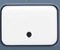# DecimalsBasics
• Decimals are based on the number ten.
• The basic units of the decimal system are 0 1 2 3 4 5 6 7 8 9 10.
• Many arithmetic problems produce an answer in decimal form.
• Zero is often used as a placeholder to the left of the decimal point —> 0.5 = .5
• There is an invisible decimal point to the right of any whole number —> 7. = 7

When asked to convert π, a fraction, or a square root to decimal form, use the toggle button.is the decimal point command.

To convert a decimal to percent, move the decimal point two places to the right; this maneuver is the same as multiplying the decimal by 100.
0.07 → 7.% = 7%
0.07 × 100 = 7%
0.70 → 70.% = 70%
0.70 × 100 = 70%

To convert percent to a decimal, move the invisible decimal point two places to the left; this maneuver is the same as dividing the percent by 100.
0.07 ← 7.% = 7%
7 ÷ 100 = 0.07
0.70 ← 70.% = 70%
70 ÷ 100 = 0.70

The decimal system positions digits as wholes (tens, hundred, thousands, etc.) and parts (tenths, hundredths, thousandths, etc.) of ten.

Positions
2146.8

thousands

2146.87
↑
hundreds

2146.873
”””
”””tens

2146.873
””””’
””””’ones

2146.873
””””””’
””””””’tenths

2146.873
””””””””
””””””””hundredths

2146.873
””””””””””
””””””””””thousandths

Question
Convert 0.08 to percent.

8%

To convert a decimal to percent, move the decimal point two places to the right.

0.08 → 8.% = 8%

Question
Convert 0.85 to percent.

85%

To convert a decimal to percent, move the decimal point two places to the right.

0.85 → 85.% = 85%

Question
Convert 6% to a decimal.

0.06

To convert percent to a decimal, move the invisible decimal point two places to the left.

0.06 ← 6.% = 6%

Question
Convert 65% to a decimal.

0.65

To convert percent to a decimal, move the invisible decimal point two places to the left.

0.65 ← 65.% = 65%

Question

What is 6.93 + 0.25?

7.18

Input Display Commentblinker clears screen93 + 025 6.93+0.25 Addition7.18 Answer
###### Decimals

Question

What is 6.93 – 0.25?

6.68

Input Display Commentblinker clears screen93 – 025 6.93-0.25 Subtraction6.68 Answer
###### Decimals

Question

What is 6.93 × 0.25?

1.7325

Input Display Commentblinker clears screen93 × 025 6.93*0.25 Multiplication1.7325 Answer
###### Decimals

Question

What is 6.93 ÷ 0.25?

27.72

Input Display Commentblinker clears screen93 ÷ 025 6.93÷0.25 Division27.72 Answer
###### Decimals

Question
Round the previous answer to the nearest tenth.

27.7

See Rounding.
27.72  27.7

Practice – Questions
1.  Convert 9% to a decimal.

2.  Convert 95% to a decimal.

3.  Convert 0.04 to percent.

4.  Convert 0.45 to percent.

5.  Convert 0.455 to percent.

6.  What is 3.94 + 0.33?

7.  What is 3.94 – 0.33?

8.  What is 3.94 × 0.33?

9.  What is 3.94 ÷ 0.33?

10.  With reference to Question 9, round your answer to the nearest hundredth.

1.  0.09

2.  0.95

3.  4%

4.  45%

5.  45.5%

6.  4.27

7.  3.61

8.  1.3002

9.  11.93939394

10.  11.94